# Online Class: Pre-Algebra 101\$50.00
no certificate
- OR -
\$75.00
with CEU Certificate*
Start Right Now!
Taking multiple courses? Save with our platinum program.

• 15
Lessons
• 31
Exams &
Assignments
• 6,326
Students
have taken this course
• 14
Hours
average time
• 1.4
CEUs## Course Description

An understanding of algebra is a foundational skill to virtually all topics in higher-level mathematics, and it is useful in science, statistics, accounting, and numerous other professional and academic areas. This course builds a bridge between simple whole-number arithmetic operations, such as addition, subtraction, multiplication, and division, and algebra.

This course begins by reviewing the basic arithmetic operations and their mutual relationships, and it then goes on to develop an understanding of fractions and how to perform operations on them. Exponents and their properties are then derived, and radicals (and other roots) are presented.

This course shows how exponents and roots are related, as well as how these are related to multiplication and division. Throughout this first portion of the course, the use of symbols in place of numbers is introduced and developed, leading to an investigation into variables and algebraic relations in the latter portion of the course.

This course also describes how to graph algebraic relations and how to solve simple linear equations. Finally, the course applies these various concepts to geometry by considering some of the characteristics of triangles, rectangles, and circles, and it also applies them to basic probability and statistics.

Thus, this course not only provides a fundamental set of algebra skills, but it demonstrates how these skills can be used to study various problems that have real-world applications.
• Completely Online
• Self-Paced
• 6 Months to Complete
• Start Anytime
• PC & Mac Compatible
• Android & iOS Friendly
• Accredited CEUs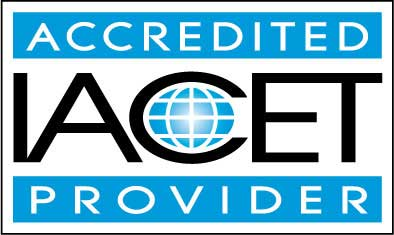## Course Lessons

Average Lesson Rating: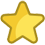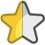#### Lesson 1 - Review of Basic Operations

This lesson reviews the basic operations and some associated ideas, setting the stage for learning about fractions, the first main topic of the course. 18 Total Points
•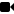Lesson 1 Video A
•Lesson 1 Video B : How to Take an Exam
•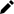Review 5 Practice Worksheets
•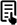Review Article: Introduction to Negative Numbers
•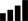Take Survey: Reasons for Taking this Course
•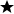Complete: Introduction Assignment
•Complete: Lesson 1 Exercises
•Complete Exam: Lesson 1 - Review of Basic Operations

#### Lesson 2 - Fractions I

This lesson provides a foundation for understanding how to add, subtract, multiply, and divide fractions--a topic to which we will turn in the next lesson. 19 Total Points
•Lesson 2 Video
•Review 5 Practice Worksheets
•Complete: Lesson 2 Exercises
•Complete Exam: Lesson 2 – Fractions I

#### Lesson 3 - Fractions II

In this lesson, we apply our fundamental understanding of fractions from the previous lesson to the basic arithmetic operations. 18 Total Points
•Lesson 3 Video
•Review 5 Practice Worksheets
•Complete: Lesson 3 Exercises
•Complete Exam: Lesson 3 – Fractions II

#### Lesson 4 - Decimals, Percents, and Ratios

In the previous two lessons, we discussed fractions at length. As you have probably noticed, however, most basic calculators (as well as many more-advanced calculators) do not deal with fractions. 20 Total Points
•Lesson 4 Video
•Review 5 Practice Worksheets
•Complete: Lesson 4 Exercises
•Complete Exam: Lesson 4 – Decimals, Percents, and Ratios

#### Lesson 5 - Exponents I

In this lesson, we introduce exponents, which are a way to represent repeated multiplication (just as multiplication is a way to represent repeated addition). 18 Total Points
•Lesson 5 Video
•Review 5 Practice Worksheets
•Review Article: The Law of Exponents
•Complete: Lesson 5 Exercises
•Complete Exam: Lesson 5 – Exponents I

#### Lesson 6 - Exponents II

Specifically, this lesson considers rules for multiplying and dividing expressions with exponents, and it also explores the meaning of negative exponents. 19 Total Points
•Lesson 6 Video
•Review 5 Practice Worksheets
•Complete: Lesson 6 Exercises
•Complete Exam: Lesson 6 – Exponents II

#### Lesson 7 - Radicals I

By the end of this lesson, you should be able to handle numbers raised to any exponent, and you will also be equipped to study arithmetic operations on radical expressions in the next lesson. 18 Total Points
•Lesson 7 Video
•Review 5 Practice Worksheets
•Complete: Lesson 7 Exercises
•Complete Exam: Lesson 7 – Radicals I

#### Lesson 8 - Radicals II

The mathematical tools we study in this lesson allow us to at least partially evaluate radical expressions without losing exactness in the result (unlike what would happen if we simply used a calculator). 16 Total Points
•Lesson 8 Video
•Review 5 Practice Worksheets
•Review Article: Operations With Radicals
•Complete: Lesson 8 Exercises
•Complete Exam: Lesson 8 – Radicals II

#### Lesson 9 - Variables and Algebraic Relations

This lesson focuses mainly on the concepts associated with these topics and lays a foundation for studying in later lessons how to graph relations and solve simple equations. 14 Total Points
•Lesson 9 Video
•Review 5 Practice Worksheets
•Complete: Lesson 9 Exercises
•Complete Exam: Lesson 9 Variables and Algebraic Relations

#### Lesson 10 - Graphing Algebraic Relations

In this lesson, we learn how to graph simple and moderately complicated algebraic relations. 10 Total Points
•Lesson 10 Video
•Review 5 Practice Worksheets
•Complete Exam: Lesson 10 ? Graphing Algebraic Relations

#### Lesson 11 - Solving Linear Equations

In this lesson, we learn how to manipulate equations by performing operations on both sides of the equation 14 Total Points
•Lesson 11 Video
•Review 5 Practice Worksheets
•Complete: Lesson 11 Exercises
•Complete Exam: Lesson 11 Solving Linear Equations

#### Lesson 12 - Geometry I: Rectangles and Triangles

In this lesson, we take what we have learned about numbers, operations, and variables and apply it to simple geometry. 13 Total Points
•Lesson 12 Video
•Review 5 Practice Worksheets
•Complete: Lesson 12 Exercises
•Complete Exam: Lesson 12 – Geometry I: Rectangles and Triangles

#### Lesson 13 - Geometry II

In this lesson, we apply our basic algebra skills to the properties of circles. We look at the circumference and area of a circle, and we introduce the number pi (π) 14 Total Points
•Lesson 13 Video
•Review 4 Practice Worksheets
•Review Article: Area of a Circle
•Complete: Lesson 13 Exercises
•Complete Exam: Lesson 13 – Geometry II

#### Lesson 14 - Introduction to Probability

In this lesson, we take some of the principles we have learned in previous lessons and apply them to some simple concepts and problems in probability. 15 Total Points
•Lesson 14 Video
•Review 5 Practice Worksheets
•Complete: Lesson 14 Exercises
•Complete Exam: Lesson 14 Introduction to Probability

#### Lesson 15 - Introduction to Statistics

The final lesson of this course looks at statistics, which is a subject that is closely related to probability. 235 Total Points
•Lesson 15 Video
•Review 5 Practice Worksheets
•Take Poll: End of Course Poll
•Take Survey: Program Evaluation Follow-up Survey (End of Course)
•Complete: Lesson 15 Exercises
•Complete Exam: Lesson 15 Introduction to Statistics
•Complete: The Final Exam
461
Total Course Points

## Learning Outcomes

By successfully completing this course, students will be able to:
• Describe basic operations or numbers and signs.
• Solve simple to complex fraction problems.
• Solve problems involving decimals, percents, and ratios.
• Solve problems involving exponents.
• Summarize variables and algebraic relations.
• Graph algebraic relations.
• Solve linear equations.
• Solve problems involving basic geometry, rectangles and triangles.
• Solve probability and statistics problems, and
• Demonstrate mastery of lesson content at levels of 70% or higher.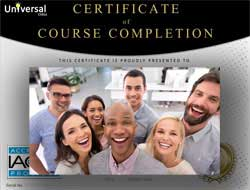• Document Your Lifelong Learning Achievements
• Earn an Official Certificate Documenting Course Hours and CEUs
• Verify Your Certificate with a Unique Serial Number OnlineCourse Title: Pre-Algebra 101
Course Number: 9770449
Lessons Rating: 4.5 / 5 Stars (2,103 votes)
Languages: English - United States, Canada and other English speaking countries
Category:
Course Type: High School Level (Self-Paced, Online Class)
CEU Value: 1.4 IACET CEUs (Continuing Education Units)
CE Accreditation: Universal Class, Inc. has been accredited as an Authorized Provider by the International Association for Continuing Education and Training (IACET).
Assessment Method: Lesson assignments and review exams
Course Author: Dr. Jeffrey Clark, Ph.D.
Instructor: April Cordry-Moore
Syllabus: View Syllabus
Duration: Continuous: Enroll anytime!
Course Fee: \$50.00 (no CEU Certification) || with Online CEU Certification: \$75.00

Course Only
One Course
No Certificate / No CEUs
\$50
for 6 months

Billed once
This course only
 Includes certificate X Includes CEUs X Self-paced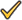Instructor supportTime to complete 6 months No. of courses 1 course
Certificate Course
One Course
Certificate & CEUs
\$75
for 6 months

Billed once
This course only
 Includes certificateIncludes CEUsSelf-pacedInstructor supportTime to complete 6 months No. of courses 1 course
Platinum YearlyALL COURSES
Certificates & CEUs
\$189
per year
You save 50%!
Billed once
Includes all 500+ courses
 Includes certificateIncludes CEUsSelf-pacedInstructor supportTime to complete 12 Months No. of courses 500+
Platinum Monthly
ALL COURSES
Certificates & CEUs
\$69 first month
\$29.00 / each consecutive month thereafter

Billed monthly
Includes all 500+ courses
 Includes certificateIncludes CEUsSelf-pacedInstructor supportTime to complete Monthly No. of courses 500+

## Student Testimonials

• "What was most helpful were the detailed course lessons and the examples provided. Instructor was very knowledgeable and was very good about giving detailed feedback to correct any mistakes or misunderstandings." -- William Hunter R.
• "I enjoyed this course. I'm also glad I could get on this course as often as I could. I liked the 5 exercises before the assignments in each chapter, plus the videos helped. The break down of the material was good too. Also I noticed that the assignments and the exercises addressed my working knowledge of my work and the test addressed my verbal knowledge, which is good. I notice that I have some problems with verbal knowledge. This has to do with me and maybe a health problem. I'm glad to know this. It tells me what to work on." -- Debbie E.
• "I just want to say express my sincere thank to the organizers and my instructor for being available for me. I learned a lot." -- Richard A.
• "What I liked most were the tests and assignments. It evaluates my knowledge....My teacher did a great work." -- Sunghee M.